# 卷积神经网络 + 机器视觉： L3_Loss Functions and Optimization (斯坦福CS231n）

### 前一課堂筆記連結：

1. 定义一个 Loss Function 用来量化一个训练集中“不满意的得分”的水平，简单说就是好与不好（0与1）该以哪里作为临界点。
2. 需要一个方法用来找出 Loss Function 里面的参数，能够让整体 Loss Function 得出来的值达到最小化。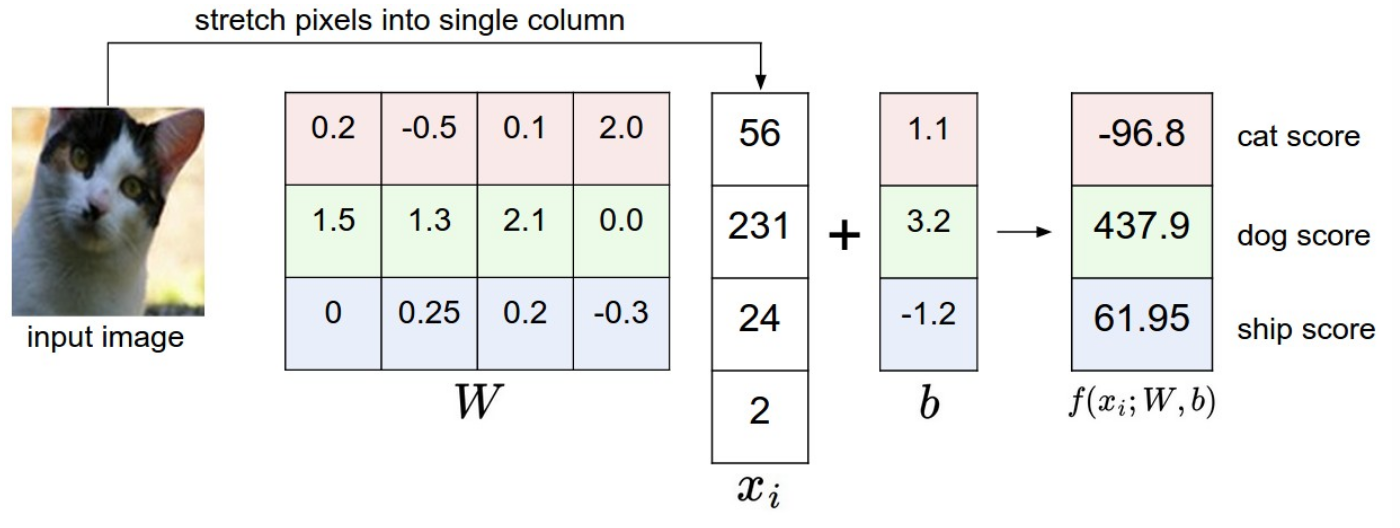-- 第一步，把一张图片的全部 pixels 拉直成为一个只有一行的矩阵，并且我们命名为数据集 Xi
-- 第二步，接着用权重矩阵与被拉长成一条的矩阵做 dot product得出每个 pixel 的重要性评比
-- 第三步，加上一个修正量目的是为了更好的让分类器能够分辨哪些零散的数据是属于哪一个区域的，提升划线区分两种不同类别的东西的操作性。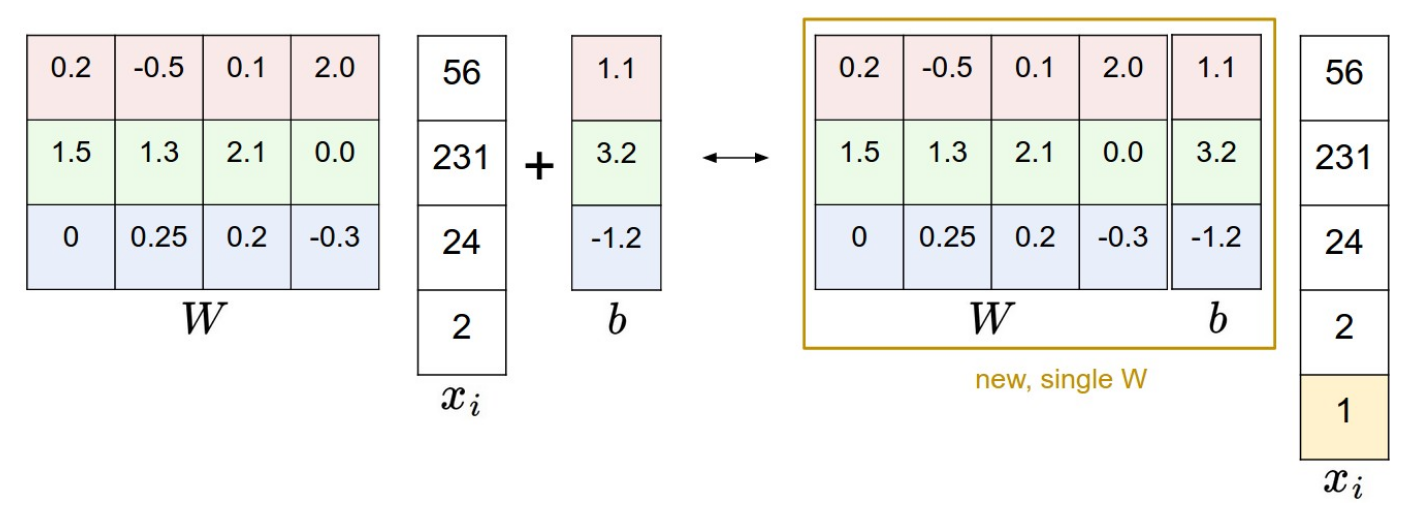Loss Function 的重要性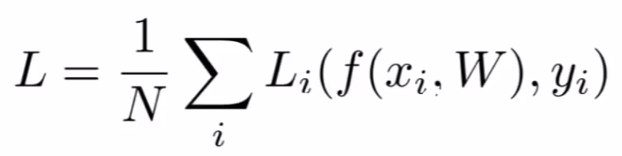x 是一张图片被拉直后做成的原始数据输入于此，是预测位置数据的依据；
y 是被线性分选器（linear classifier）预测出来的预测值。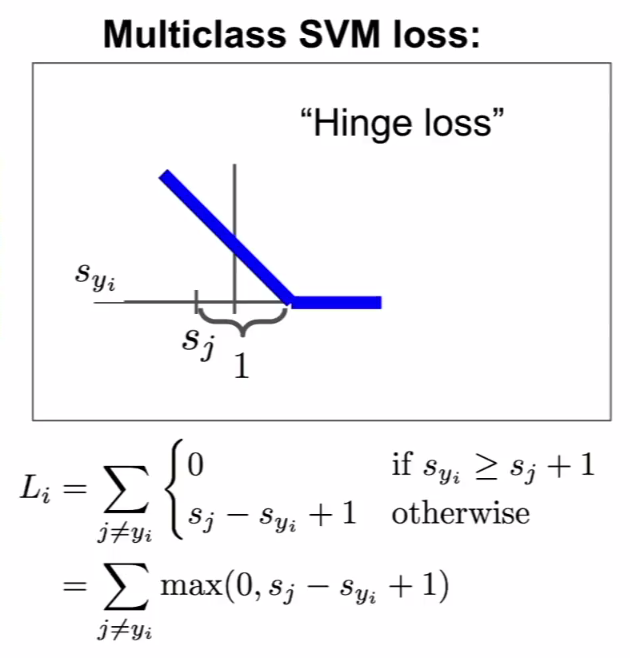Hinge Loss

猫的图片 汽车的图片 青蛙的图片

-3.1

1. Loss Function for cat: max(0, 5.1-3.2+1) + max(0, -1.7-3.2+1) = 2.9
2. Loss Function for car: max(0, 1.3-4.9+1) + max(0, 2.0-4.9+1) = 0
3. Loss Function for frog: max(0, 2.2-(-3.1)+1) + max(0, 2.5-(-3.1)+1) = 12.9
4. Entire Loss: (2.9 + 0 + 12.9)/3 = 5.27
** 补充：max() 的功能是比较 () 里面的数字，哪一个最大就选择哪一个作为答案。This triangle delta is some fixed margin indicating that the score of the correct labeled object should be higher at least THIS amount of value than the rests of the error classes so that there would be no loss. If we further stretch out this formula to be an illustrative line, it goes like this: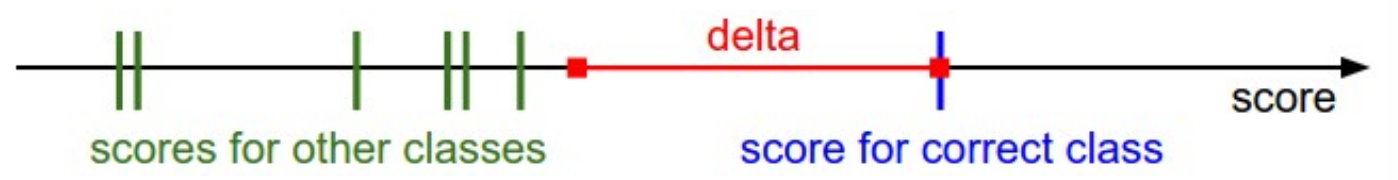>>> import numpy as np
>>> def Li_vectorized(x, y, W):
scores = W.dot(x)
margins = np.maximum(0, scores - scores[y] + delta)
margins[y] = 0
loss_i = np.sum(margins)
retrun loss_i


Special case 1:

Special case 2:

Regularization

Although linear classifier looks good when we gain the perfect Weight value, this W is still not the unique answer. We can simply multiply its value originally found as the perfect one to fit the dataset to gain the other one. Therefore, this Regularization term helps to set a preference for a certain set of weights W over others to remove this ambiguity.

Re 有很多方法，这边就只介绍 L2 Regularization (Weight Decay)。公式如下：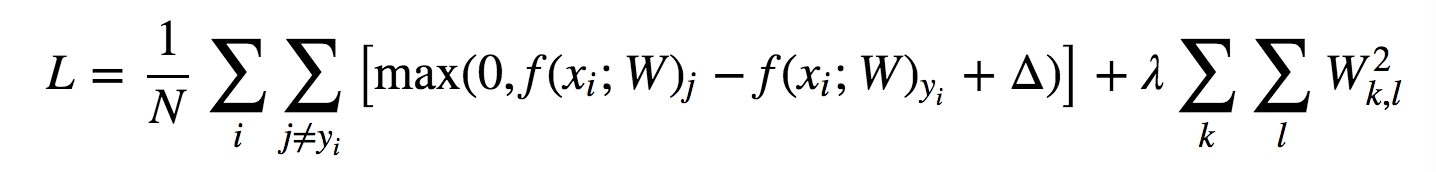The other classifier: Softmax classifier
This is similar to the output treatment by SVM. But Softmax's output is a bit more intuitive and has a probabilistic interpretation inside the formula. The loss function looks like this: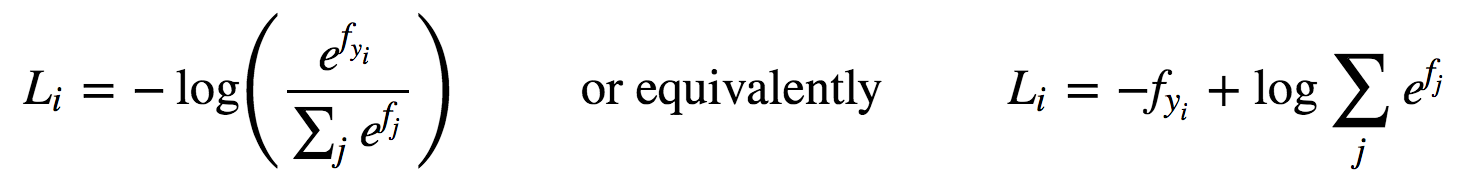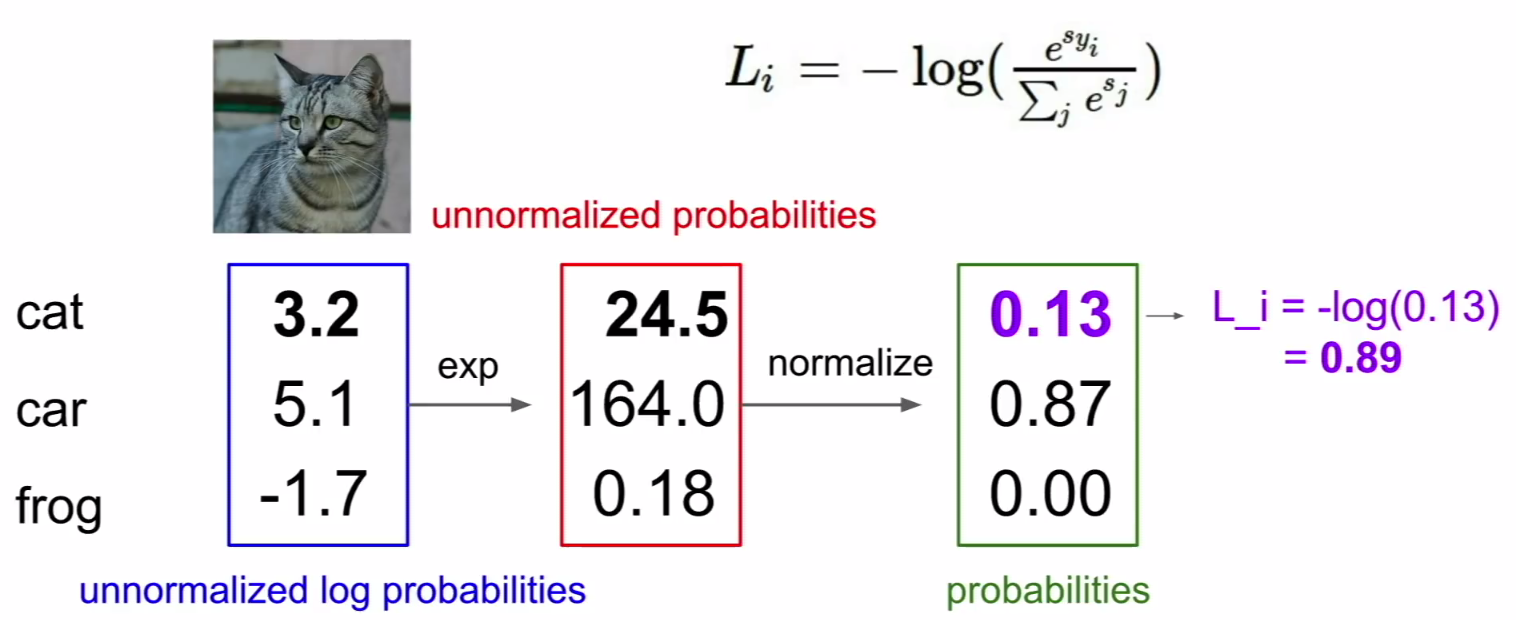1. cat：exp(3.2) = 24.5 ; 24.5/(24.5+164.0+0.18) = 0.13 ; -log(0.13) = 0.89
2. car: exp(5.1) = 164.0 ; 164.0/(24.5+164.0+0.18) = 0.87 ; -log(0.87) = 0.06
3. frog: exp(-1.7) = 0.18 ; 0.18/(24.5+164.0+0.18) = 0 ; ...

https://blog.csdn.net/u010976453/article/details/78488279

Comparison of these two methods above

o SVM Loss: 更趋近于让原本数据之间的差异保留原汁原味，甚至我们可以通过一些手段把原本就差很大的间距提升到更大。换言之，这个方法只能够基于数据本身的差异上 ”加油添醋“ ，跟现实世界的贫富差距有异曲同工之妙。

o Softmax Loss：得出来的结果有是有一个区间限制的，只能在 0～1 之间，不论原本彼此差别多么的大，最终都要把差距按照公式定义的比例压缩到这个区间里，因此这个方法强调的是如何让有差距的数据往 0 或者 1 的两极方向跑，借由让数据集分化去 0 或者 1 的一端，达到最终目的。

Optimization

Strategy #1. Random search, a very bad idea though... here are the lines of code:

>>> bestloss = float('inf')        # python assigns the highest possible float value
>>> for num in xrange(1000):
W = np.random.randn(10, 3073) * 0.0001        # to generate random parameters
loss = L(X_train, Y_train, W)        # get the loss over the entire training set
if loss < bestloss:
bestloss = loss
bestW = W
print('in attempt \$d the loss was %f, best %f' %(num, loss, bestloss))


Strategy #2. Follow the slope

>>> while True:        # in a real graph, we use for loop to run the model typically
weights_grad = evaluate_gradient(loss_fun, data, weights)
weights += -step_size * weights_grad        # perform parameter update
# step_size is also a hyperparameter used to define how fast the loop will get closed to the target.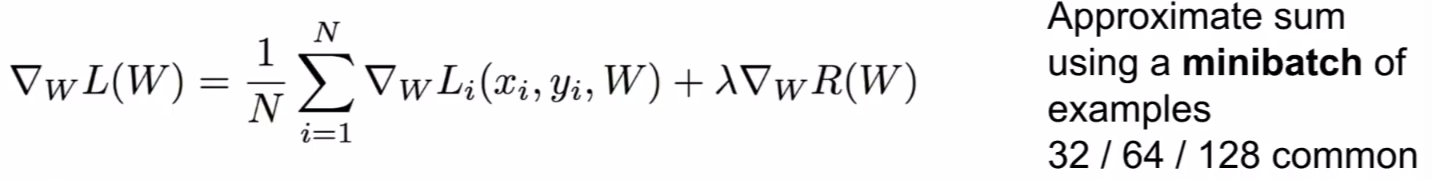In every iteration, we sample some small sets of training samples called minibatch to compute and estimate the full sum and the true gradient. The code goes like this:

>>> while True:
data_batch = sample_training_data(data, 256)
# sample 256 exampels
weights_grad = evaluate_gradient(loss_fun, data_batch, weights)
weights += -step_size * weights_grad        # perfrom parameter update


The other earlier example approaches to recognize the image:
- Histogram of Oriented Gradients (HoG): Divide image into 8*8 pixel regions and quantize edge of the regions direction into 9 bins.
- Color Histogram
- Bag of words, the inspiration from nature language processing. the logic here is to count the difference of the characteristics in different images as a paragraph. but there are not vocabulary to describe the image understood by a computer.

Image features vs ConvNets
Get an image >>> feature extraction >>> 10 numbers giving scores for classes >>> training >>> convolutional network application >>> output.

The extracted features from the images would be the fixed block that remains unchanged during the training. The only thing changed is the parameters applied in the linear classifier set to fit the minimum loss.

When we talk about CNN, it is quite similar to the earlier approach. But CNN learn the features directly from the data instead of making visual words first.